Courses

# Vogel’s Approximation Method (VAM) - Business Mathematics and Statistics B Com Notes | EduRev

Created by: Arshit Thakur

## B Com : Vogel’s Approximation Method (VAM) - Business Mathematics and Statistics B Com Notes | EduRev

The document Vogel’s Approximation Method (VAM) - Business Mathematics and Statistics B Com Notes | EduRev is a part of the B Com Course Business Mathematics and Statistics.
All you need of B Com at this link: B Com

Vogel’s Approximation Method (VAM)

The steps involved in this method for finding the initial solution are as follows.

Step 1  Find the penalty cost, namely the difference between the smallest and next smallest costs in each row and column.

Step 2  Among the penalties as found in Step(1) choose the maximum penalty.
If this maximum penalty is more than one (i.e., if there is a tie) choose any one arbitrarily.

Step 3  In the selected row or column as by Step(2) find out the cell having the least cost. Allocate to this cell as much as possible depending on the capacity and requirements.

Step 4  Delete the row or column which is fully exhausted. Again, compute the column and row penalties for the reduced transportation table and then go to Step (2). Repeat the procedure until all the rim requirements are satisfied.

Note  If the column is exhausted, then there is a change in row penalty and vice versa.

Example 3.5  Find the initial basic feasible solution for the following transportation problem by VAM.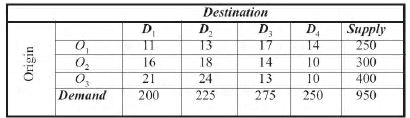Solution  Since Σai = Σbj = 950 the problem is balanced and there exists a feasible solution to the problem.
First, we find the row Σ column penalty PI as the difference between the least and the next least cost. The maximum penalty is 5. Choose the first column arbitrarily.

In this column, choose the cell having the least cost name (1, 1). Allocate to this cell with minimum magnitude (i.e.(250, 200) = 200.) This exhausts the first column.
Delete this column. Since a column is deleted, then there is a change in row penalty PII and column penalty PII remains the same. Continuing in this manner, we get the remaining allocations as given in the following table below.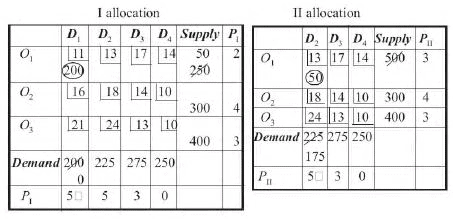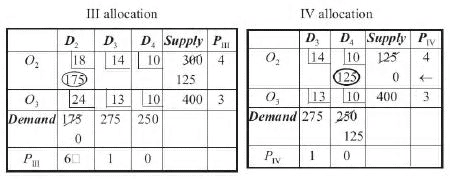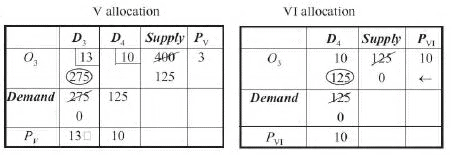Finally, we arrive at the initial basic feasible solution which is shown in the following table.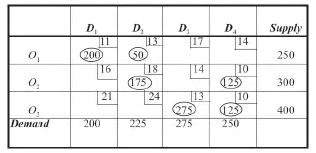There are 6 positive independent allocations which equals to m + n –1 = 3 + 4 – 1. This ensures that the solution is a non-degenerate basic feasible solution.

∴ The transportation cost

= 11 × 200 + 13 × 50 + 18 × 175 + 10 × 125
+ 13 × 275 + 10 × 125 = Rs 12,075.

Example 3.6  Find the initial solution to the following TP using VAM.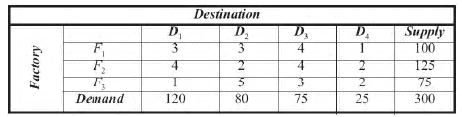Solution  Since Σai = Σ bj the problem is a balance TP. Hence, there exists a feasible solution.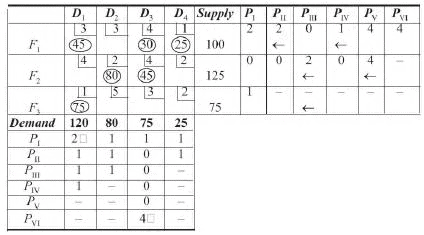Finally, we have the initial basic feasible solution as given in the following table.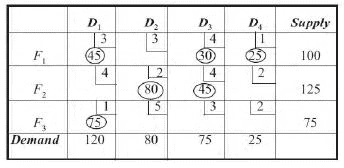There are 6 independent non-negative allocations equal to m]n[1 \3]4[1\6. This ensures that the solution is non-degenerate basic feasible.

∴  The transportation cost = 3 × 45 + 4 × 30 + 1× 25 + 2 × 80 + 4 × 45 + 1 + 75
= 135 + 120 + 25 + 160 + 180 + 75
= Rs 695

Offer running on EduRev: Apply code STAYHOME200 to get INR 200 off on our premium plan EduRev Infinity!

122 videos|142 docs

,

,

,

,

,

,

,

,

,

,

,

,

,

,

,

,

,

,

,

,

,

;